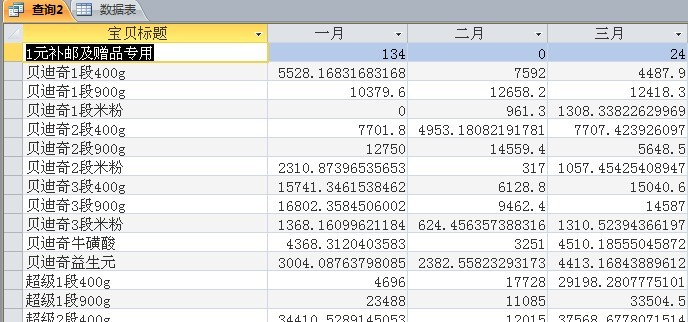# access 查询修改字段怎么写？

ID 标题

1 【买4赠1本品 免邮】飞鹤飞帆1段婴幼儿配方奶粉900g 听装
2 飞鹤飞帆2段婴幼儿配方奶粉三联包1.2kg特惠装（不参加买送）
3 【买4赠1本品 免邮】飞鹤飞帆2段婴幼儿配方奶粉400g盒装 湿法
4 【聚】飞鹤飞帆抵抗力婴幼儿配方奶粉900g听装
5 【买4赠1本品 免邮】飞鹤飞帆新3段抵抗力婴幼儿奶粉 900g听

ID 标题

1 飞帆1段900g
2 飞帆2段三联包
3 飞帆2段400g
4 飞帆抵抗力900g
5 飞帆抵抗力900g

...全文
517 点赞 收藏 22

22 条回复

ZJCCCC 2013-07-08

wwwwb 2013-07-08
SELECT [宝贝标题], sum(IIf(month([订单创建时间])=1,[总金额],0)) AS 一月, sum(IIf(month([订单创建时间])=2,[总金额],0)) AS 二月, sum(IIf(month([订单创建时间])=3,[总金额],0)) AS 三月, sum(IIf(month([订单创建时间])=4,[总金额],0)) AS 四月, sum(IIf(month([订单创建时间])=5,[总金额],0)) AS 五月, sum(IIf(month([订单创建时间])=6,[总金额],0)) AS 六月, sum(IIf(month([订单创建时间])=7,[总金额],0)) AS 七月, sum(IIf(month([订单创建时间])=8,[总金额],0)) AS 八月, sum(IIf(month([订单创建时间])=9,[总金额],0)) AS 九月, sum(IIf(month([订单创建时间])=10,[总金额],0)) AS 十月, sum(IIf(month([订单创建时间])=11,[总金额],0)) AS 十一月, sum(IIf(month([订单创建时间])=12,[总金额],0)) AS 十二月 FROM 数据表 where 平台名称＝“分销” GROUP BY [宝贝标题];

ZJCCCC 2013-07-08

WWWWA 2013-07-05

ZJCCCC 2013-07-05SELECT [宝贝标题],
sum(IIf(month([订单创建时间])=1,[总金额],0)) AS 一月,
sum(IIf(month([订单创建时间])=2,[总金额],0)) AS 二月,
sum(IIf(month([订单创建时间])=3,[总金额],0)) AS 三月,
sum(IIf(month([订单创建时间])=4,[总金额],0)) AS 四月,
sum(IIf(month([订单创建时间])=5,[总金额],0)) AS 五月,
sum(IIf(month([订单创建时间])=6,[总金额],0)) AS 六月,
sum(IIf(month([订单创建时间])=7,[总金额],0)) AS 七月,
sum(IIf(month([订单创建时间])=8,[总金额],0)) AS 八月,
sum(IIf(month([订单创建时间])=9,[总金额],0)) AS 九月,
sum(IIf(month([订单创建时间])=10,[总金额],0)) AS 十月,
sum(IIf(month([订单创建时间])=11,[总金额],0)) AS 十一月,
sum(IIf(month([订单创建时间])=12,[总金额],0)) AS 十二月
FROM 数据表
GROUP BY [宝贝标题];

ACMAIN_CHM 2013-07-04

WWWWA 2013-07-04
UPDATE TT SET 标题=iif(instr(标题,'飞帆')>0 and instr(标题,'1段')>0 and instr(标题,'900g')>0,'飞帆1段900g',iif(instr(标题,'飞帆')>0 and instr(标题,'2段')>0 and instr(标题,'三联包')>0,'飞帆2段三联包',if(instr(标题,'飞帆')>0 and instr(标题,'2段')>0 and instr(标题,'400g')>0,'飞帆2段400g',iif(instr(标题,'飞帆')>0 and instr(标题,'抵抗力')>0 and instr(标题,'900g')>0,'飞帆抵抗力900g',标题))))

ZJCCCC 2013-07-04

ZJCCCC 2013-07-04

WWWWA 2013-07-04

ZJCCCC 2013-07-04

wwwwb 2013-07-04
SELECT *, iif(instr(标题,'飞帆')>0 and instr(标题,'1段')>0 and instr(标题,'900g')>0,'飞帆1段900g',iif(instr(标题,'飞帆')>0 and instr(标题,'2段')>0 and instr(标题,'三联包')>0,'飞帆2段三联包',if(instr(标题,'飞帆')>0 and instr(标题,'2段')>0 and instr(标题,'400g')>0,'飞帆2段400g',iif(instr(标题,'飞帆')>0 and instr(标题,'抵抗力')>0 and instr(标题,'900g')>0,'飞帆抵抗力900g',标题)))) as ss from tt

ZJCCCC 2013-07-04

ZJCCCC 2013-07-04

WWWWA 2013-07-04
3、建议你看看有关SQL方面的基础知识

WWWWA 2013-07-04
1、并不是所有查询都能更新的 2、更新的是基表中的数据，而不是查询出来的数据

WWWWA 2013-07-04

ZJCCCC 2013-07-04

7508

Microsoft Office Access是由微软发布的关系数据库管理系统。它结合了 MicrosoftJet Database Engine 和 图形用户界面两项特点。